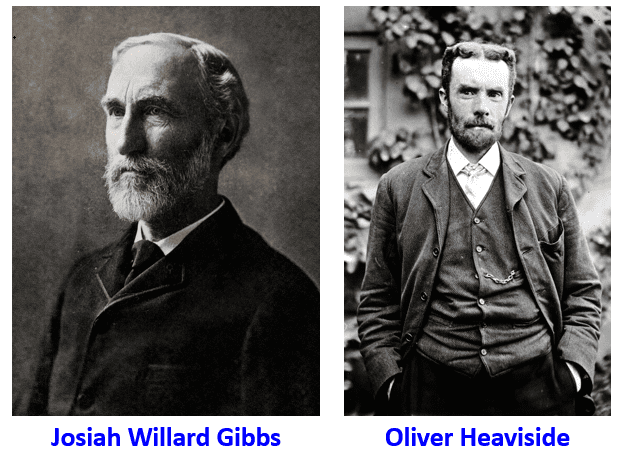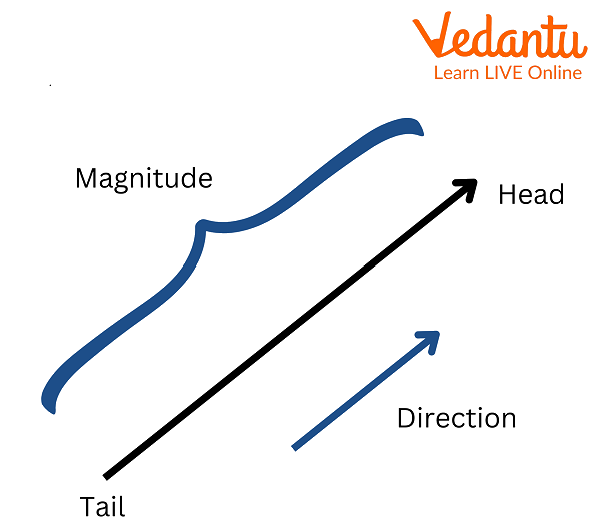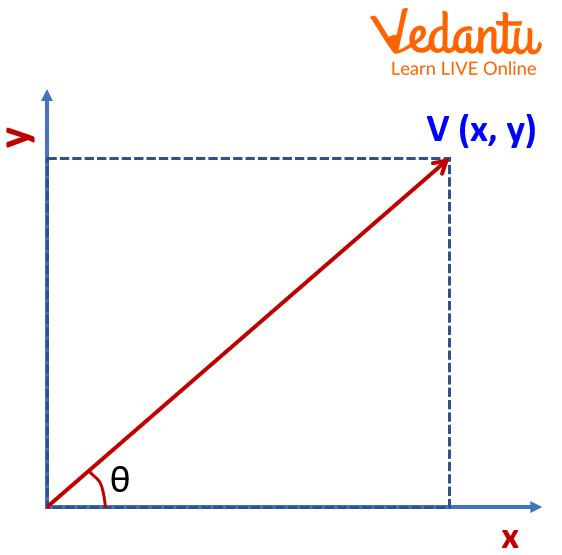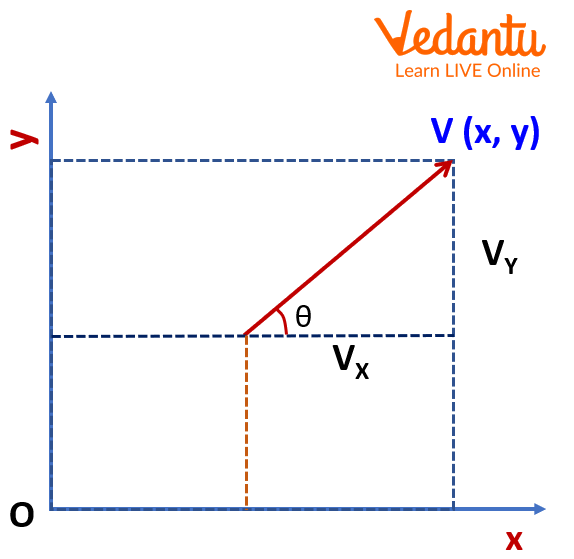Courses
Courses for Kids
Free study material
Free LIVE classes
More

# Vector## Vector: An Introduction

A vector is a quantity in physics that has both magnitude and direction. It is frequently depicted as an arrow with a length proportional to the magnitude and a direction matching the magnitude of the quantity. A vector has both magnitude and direction.

Scalars are regular quantities with a magnitude but no direction. For instance, speed (the amount of velocity), time, and mass are scalars. Examples for vectors: displacement, velocity, momentum, and acceleration are vector quantities.

## History of Inventor

Late in the 19th century, American and British physicists Josiah Willard Gibbs and Oliver Heaviside separately created vector analysis to represent the new electromagnetic principles found by Scottish scientist James Clerk Maxwell. This is when vectors first took on their current shape.Pictures of Josiah Willard Gibbs and Oliver Heaviside

## Vector Representation

How to represent a vector? A vector has a Tail as the initial point and a tip as the terminal point. You can represent the vector as $\vec a$ (vector a). The magnitude of the vector is represented by the length of the line AB denoted as $\left| a \right|$. Any line can be represented as an AB line segment but an arrow mark represents the vector $\overrightarrow {AB}$.

### Vector DiagramVector diagram

This is the vector diagram in which length is the magnitude and one end is the head and one is the tail.

## Representation of Vector Graphically

Graphically a vector is represented by an arrow. When a vector is represented visually, the length of the arrow serves as a reference for the vector's magnitude, and the arrow's direction serves as a reference for the vector's direction.

## Magnitude of a Vector

The length of a vector represents its magnitude and is denoted as $\left| a \right|$. The magnitude is always positive, it cannot be negative. This magnitude of a vector formula provides a summary of the individual vector measurements along the x, y, and z axes.

## Magnitude of a Vector Formula

If one point is the origin and another point is (x, y) of any vector $\vec a$ then we can calculate the magnitude as $\left| a \right|$=$\sqrt {{x^2} + {y^2}}$

• If both points are known $({x_1},{y_1})$and $({x_2},{y_2})$ then the magnitude is $\left| a \right|$=$\sqrt {{{({x_2} - {x_1})}^2} + ({y_2} - } {y_1}{)^2}$

• If the vector is given in $\vec a$= ${x_1}i + {y_1}j + {z_1}k$ then the magnitude $\left| a \right|$=$\sqrt {{x_1}^2 + {y_1}^2 + {z_1}^2}$

## Direction of a Vector

The direction of a vector is the slope of that vector with the horizontal axis. How to find the direction of a vector? Let’s say we have a vector starting from the origin, and the endpoint is (x, y) then we can calculate the direction by using its slope, that’s tan θ = y/x.Graph showing the direction of a vector

To calculate the angle, we use θ = tan-1 (y/x).

If two points are given for any vector $({x_1},{y_1})$and $({x_2},{y_2})$then the formula to find $(x,y)$ = $({x_2} - {x_1},{y_2} - {y_1})$and then we can use the same formula to calculate the angle θ = tan-1 (y/x).

## Components of Vector

In a two-dimensional coordinate system, the component of a vector is the x-component (x-axis)and y-component (y-axis). Vx and Vy are the components of the x-axis and y-axis for a given vector V.Graph showing the components of a vector V and O is the origin

If angle θ is formed between vector V and the x-component for any right triangle then we can use trigonometric ratios to find the components of the given vector.

• sin θ = $\dfrac{{Perpendicular}}{{Hypotenuse}}$

• cos θ = $\dfrac{{Base}}{{Hypotenuse}}$

If we use for the components Vx and Vy.:

• sin θ = $\dfrac{{{V_y}}}{V}$

Vy =V sin θ

• cos θ = $\dfrac{{{V_x}}}{V}$

Vy= V cos θ

## Interesting Facts

• Vectors are used for navigation in both the air and on boats. To establish how long they must fly before turning or landing, planes are assigned a vector to follow.

• Vector is also used in a series of instructions or mathematical assertions that arrange lines and shapes in a two-dimensional or three-dimensional space to produce vector graphics, which are computer pictures.

## Solved Problem

1. Find the magnitude of the vector $\overrightarrow {AB}$ if the initial point is (3, 6) and the endpoint is (8, 12).

Sol.

Given: Initial point (3,6) and endpoint (8, 12)

We can use the magnitude formula to solve this vector.

Magnitude formula for a given vector = $\sqrt {{{({x_2} - {x_1})}^2} + ({y_2} - } {y_1}{)^2}$

$\Rightarrow \text{Magnitude of a vector}= \sqrt {{{(8 - 3)}^2} + {{(12 - 6)}^2}}$

$\Rightarrow \text{Magnitude of a vector}=\sqrt {{{(5)}^2} + {{(6)}^2}}$

$\Rightarrow \text{Magnitude of a vector}=\sqrt {25 + 36}$

$\therefore \text{Magnitude of a vector}=\sqrt {61}$ =7.81(approx.)

Therefore, the approximate value of the magnitude of a vector is 7.81.

2. Find the direction of a vector if the initial point is (2, 6) and the endpoint is (4, 9).

Sol.

Given: Initial point is (2, 6) and the Endpoint is (4, 9).

To calculate the angle, we use θ = tan-1 (y/x).

$(x,y)$ = $({x_2} - {x_1},{y_2} - {y_1})$

$\Rightarrow (x,y)$= $(4 - 2,9 - 6)$

$\Rightarrow (x,y)$= (2,3)

$\Rightarrow$ θ = tan-1 (y/x)

$\Rightarrow$ θ = tan-1 (3/2)

$\therefore$ θ = 56.3 degrees

Hence the direction of a given vector is 56.3 degrees.

## Summary

Vector has both magnitude and direction with its help. We can use it in physics numerical problems and also in real-world scenarios. Vectors can be represented graphically or we can also find the direction if we have coordinated the points. The component of the vector will help us to show the vector on the two-dimensional coordinate system.

## List of Related Articles

• Components of Vector
• Vector Formulas
Last updated date: 27th May 2023
Total views: 3.9k
Views today: 0.01k

## FAQs on Vector

1. Which physical quantity can be called a vector?

A physical quantity which can be defined only with the direction and magnitude, is called a vector. For example, Force, displacement, and velocity are the vector quantities, whereas speed, distance are the scalar quantities.

2. What is a vector in real life?

Many real-world scenarios involving force or velocity may be applied to vectors. Take the pressures on a boat crossing a river as an illustration. A force is produced in one direction by the boat's motor and another in another by the river's stream. They are both vector forces. In Physics, we use vectors to give the direction and magnitude because it is an easier method to prove the data in graphical form instead of using numbers or other variables.

3. What is the length of a vector?

We may measure a vector's characteristic using its length, sometimes referred to as its magnitude. Simply sum up the squares of a vector's parts and take the square root of the result to get its length. If we compare any two vectors or any other quantities then the length of the vector gives us an idea of how much the magnitude is more or less. While calculating the length, always check both components of the vector. Which are represented by the vector on the x-axis and y-axis.